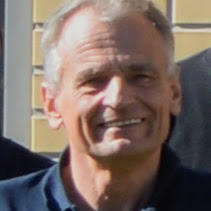• ### Marek Zaionc - Asymptotic Densities in Logic and Computability

• Wednesday, June 14, 2017 2:00 PM - 3:00 PM EDT
Haas Hall Rm. 111
Purdue University

This talk presents numerous results from the area of quantitative investigations in logic and computability. We present a quantitative analysis of random formulas or random lambda terms or random combinatory logic terms. Our main goal is to investigate likelihood of semantic properties of random computational objects. For the given logical calculus (or type theory or combinatory logic term) we investigate the proportion of the number of distinguished formulas (or types or terms) of a certain length n to the number of all formulas of such length. We are especially interested in asymptotic behavior of this fraction when n tends to innity. For the given set A of objects the limit \u03bc ( A ) if exists, is an asymptotic probability of nding formula from the class A among all formulas or may also be interpreted as the asymptotic density of the set A . Results obtained are having some philosophical flavor like for example:

1. How big is the fraction of one logic being sub-logic of the bigger one based on the same language,
2. Estimate chances that random formula is true or how big is the fraction of tautologies?
3. What are chances that random program terminates?Presented by Marek Zaionc
Professor, Theoretical Computer Science
Jagiellonian University
Krakow, Poland